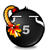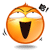好個東森，讓我們被抱怨到呆掉~~ 一__一"

主題: 好個東森，讓我們被抱怨到呆掉~~ 一__一"

1. 好個東森，讓我們被抱怨到呆掉~~ 一__一"

用公司電腦測的：

Pinging www.gamania.com [210.208.85.11] with 32 bytes of data:

Reply from 210.208.85.11: bytes=32 time=317ms TTL=247
Reply from 210.208.85.11: bytes=32 time=330ms TTL=247
Reply from 210.208.85.11: bytes=32 time=301ms TTL=247
Reply from 210.208.85.11: bytes=32 time=299ms TTL=247

Ping statistics for 210.208.85.11:
Packets: Sent = 4, Received = 4, Lost = 0 (0% loss),
Approximate round trip times in milli-seconds:
Minimum = 299ms, Maximum = 330ms, Average = 311ms

家中 SO-NET 2M/256K 測的：
PING www.gamania.com (210.208.85.11) 56(84) bytes of data.
64 bytes from www.gamania.com (210.208.85.11): icmp_seq=1 ttl=247 time=45.6 ms
64 bytes from www.gamania.com (210.208.85.11): icmp_seq=2 ttl=247 time=44.6 ms
64 bytes from www.gamania.com (210.208.85.11): icmp_seq=3 ttl=247 time=43.6 ms
64 bytes from www.gamania.com (210.208.85.11): icmp_seq=4 ttl=247 time=43.5 ms
64 bytes from www.gamania.com (210.208.85.11): icmp_seq=5 ttl=247 time=43.6 ms
64 bytes from www.gamania.com (210.208.85.11): icmp_seq=6 ttl=247 time=43.5 ms
64 bytes from www.gamania.com (210.208.85.11): icmp_seq=7 ttl=247 time=44.5 ms
64 bytes from www.gamania.com (210.208.85.11): icmp_seq=8 ttl=247 time=44.5 ms
64 bytes from www.gamania.com (210.208.85.11): icmp_seq=9 ttl=247 time=43.5 ms
64 bytes from www.gamania.com (210.208.85.11): icmp_seq=10 ttl=247 time=44.5 ms

小弟馬上寄信抱怨企~回覆時引用此文章

2. 過了尖峰時間就正常了~Pinging www.gamania.com [210.208.85.11] with 32 bytes of data:

Reply from 210.208.85.11: bytes=32 time=25ms TTL=247
Reply from 210.208.85.11: bytes=32 time=16ms TTL=247
Reply from 210.208.85.11: bytes=32 time=17ms TTL=247
Reply from 210.208.85.11: bytes=32 time=20ms TTL=247
Reply from 210.208.85.11: bytes=32 time=24ms TTL=247
Reply from 210.208.85.11: bytes=32 time=20ms TTL=247
Reply from 210.208.85.11: bytes=32 time=10ms TTL=247
Reply from 210.208.85.11: bytes=32 time=17ms TTL=247
Reply from 210.208.85.11: bytes=32 time=4ms TTL=247
Reply from 210.208.85.11: bytes=32 time=5ms TTL=247
Reply from 210.208.85.11: bytes=32 time=4ms TTL=247
Reply from 210.208.85.11: bytes=32 time=13ms TTL=247
Reply from 210.208.85.11: bytes=32 time=17ms TTL=247
Reply from 210.208.85.11: bytes=32 time=17ms TTL=247
Reply from 210.208.85.11: bytes=32 time=18ms TTL=247
Reply from 210.208.85.11: bytes=32 time=19ms TTL=247
Reply from 210.208.85.11: bytes=32 time=3ms TTL=247
Reply from 210.208.85.11: bytes=32 time=5ms TTL=247
Reply from 210.208.85.11: bytes=32 time=6ms TTL=247
Reply from 210.208.85.11: bytes=32 time=4ms TTL=247
Reply from 210.208.85.11: bytes=32 time=11ms TTL=247
Reply from 210.208.85.11: bytes=32 time=9ms TTL=247
Reply from 210.208.85.11: bytes=32 time=22ms TTL=247
Reply from 210.208.85.11: bytes=32 time=7ms TTL=247

Ping statistics for 210.208.85.11:
Packets: Sent = 24, Received = 24, Lost = 0 (0% loss),
Approximate round trip times in milli-seconds:
Minimum = 3ms, Maximum = 25ms, Average = 13ms回覆時引用此文章

3. 大哥...您這還算小問題...
我是東森老客戶，從單向到今天雙向，真的是越來越爛，今天連榮總竟然連不上去，掛個free proxy，立刻連上去•東森的網管技術也真爛，連個proxy都管不好，這個月內應該會退掉了，一直是嫌麻煩，老婆閨房不喜歡陌生人進入，才延誤至今...回覆時引用此文章

• 不可以發表新主題
• 不可以回覆文章
• 不可以上傳附加檔案
• 不可以編輯自己的文章
•